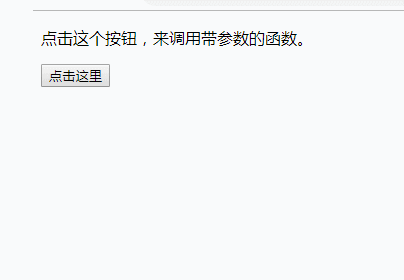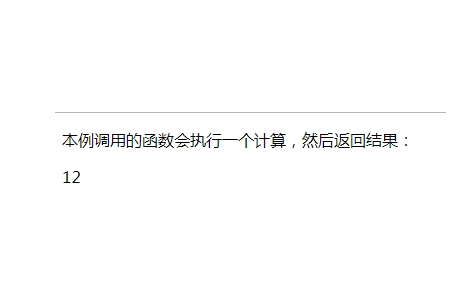# JavaScript 函数前端控 更新时间：2019-02-12 22:02:23

JavaScript 函数

```<script>
function myFunction()
{
}
</script>```

JavaScript 函数语法

```function functionname()
{
// 执行代码
}```

`myFunction(argument1,argument2)`

```function myFunction(var1,var2)
{

}```

## 实例

```<p>点击这个按钮，来调用带参数的函数。</p>
<button onclick="myFunction('小明','18')">点击这里</button>
<script>
function myFunction(name,age){
document.write(name +"是一个"+age+"岁的男孩")
}
</script>```## 实例

```<p>请点击其中的一个按钮，来调用带参数的函数。</p>
<button onclick="myFunction('小明','男孩')">点击这里</button>
<button onclick="myFunction('小红','女孩')">点击这里</button>
<script>
function myFunction(name,sex)
{
document.write(name +"是一个"+sex)
}
</script>``````function myFunction()
{
var x=5;
return x;
}```

`var myVar=myFunction();`

myVar 变量的值是 5，也就是函数` "myFunction()" `所返回的值。

`document.getElementById("demo").innerHTML=myFunction();`

"demo" 元素的 innerHTML 将成为 5，也就是函数 `"myFunction()" `所返回的值。

## 实例

```<script>
function myFunction(a,b){
return a*b;
}
document.getElementById("demo").innerHTML=myFunction(4,3);
</script>``````function myFunction(a,b){
if (a>b)
{
return;
}
x=a+b;
}```

JavaScript 变量的生存期

JavaScript 变量的生命期从它们被声明的时间开始。

`carname="Volvo";`

```var var1 = 1; // 不可配置全局属性
var2 = 2; // 没有使用 var 声明，可配置全局属性
console.log(this.var1); // 1
console.log(window.var1); // 1
delete var1; // false 无法删除
console.log(var1); //1
delete var2;
console.log(delete var2); // true
console.log(var2); // 已经删除 报错变量未定义```

php中文网
phpstudy

QQ交流群